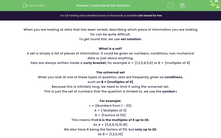# Understand Set Notation

In this worksheet, students will practise using simple set notation.Key stage:  KS 4

Year:  GCSE

GCSE Subjects:   Maths

GCSE Boards:   AQA, Eduqas, Pearson Edexcel, OCR,

Curriculum topic:   Probability

Curriculum subtopic:   Probability Combined Events and Probability Diagrams

Difficulty level:#### Worksheet Overview

When you are looking at data that has been sorted, describing which piece of information you are looking for can be quite difficult.

To get round this, we use set notation.

What is a set?

A set is simply a list of pieces of information. It could be given as numbers, conditions, non-numerical data or just about anything.

Sets are always written inside a curly bracket, for example A = {1,2,3,4,5,6} or B = {multiples of 4}

The universal set

When you look at one of these types of question, sets are frequently given as conditions,

such as B = {multiples of 4}.

Because this is infinitely long, we need to limit it using the universal set.

This is just the set of numbers that the question is limited to, we use the symbol ε

For example:

ε = {Numbers from 1 - 20}

A = { Multiples of 3}

B = {Factors of 50}

This means that A is the multiples of 3 up to 20.

So A = {3,6,9,12,15,18}

We also have B being the factors of 50, but only up to 20.

So B = {1,2,5,10}

What other notation do we need?

There are a number of set notations that you need to know for GCSE maths.

Intersection: This would be written as A∩B

This means all the values that are in A and B.

Union: This would be written as A∪B

This means all the values that are in A or B.

Not: This would be written as A'

This means all the values that are not in A.

Example:

ε = {Numbers from 1 - 20}

A = { Multiples of 2}

B = {Factors of 50}

Find the numbers that satisfy:

A∩B

These are the numbers that satisfy both set A and B, and that are 20 or less.

The only numbers that are both a factor of 50 and a multiple of 2, as well as being 20 or less are 2 and 10.

A∪B

This means we want all the numbers that are either a multiple of 2 or a factor of 50 (but still under the limit of 21).

This gives us the numbers: 1, 2, 3, 4, 5, 6, 8, 9. 10, 12, 14, 16, 18, 20

A'

Not A means all the numbers that aren't in set A.

This means the following numbers: 1, 3, 5, 7, 9, 11, 13, 15, 17, 19

There's a lot to remember here, so let's test it out in some questions.

### What is EdPlace?

We're your National Curriculum aligned online education content provider helping each child succeed in English, maths and science from year 1 to GCSE. With an EdPlace account you’ll be able to track and measure progress, helping each child achieve their best. We build confidence and attainment by personalising each child’s learning at a level that suits them.

Get started